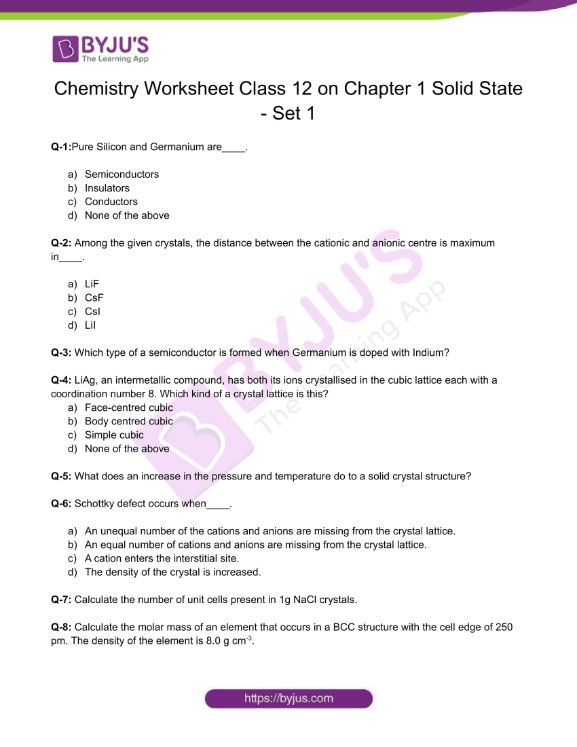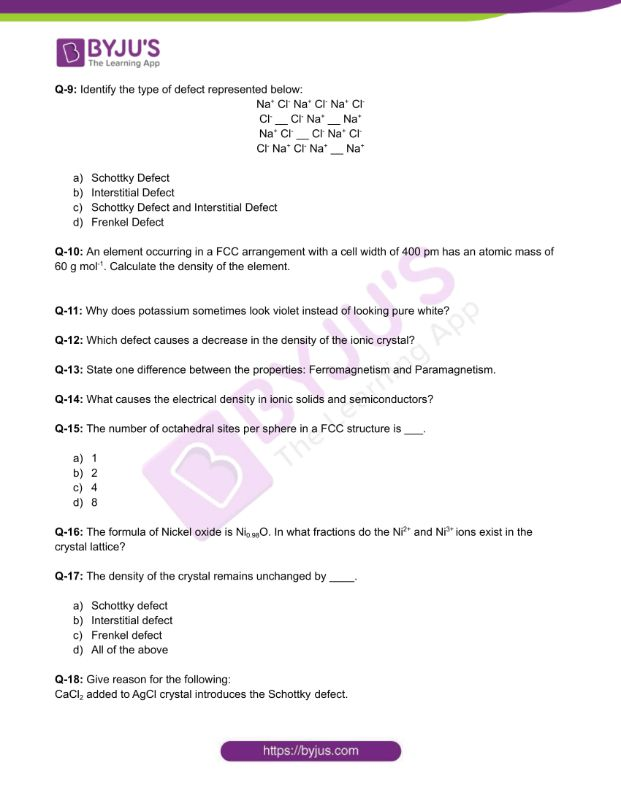Checkout JEE MAINS 2022 Question Paper Analysis : Checkout JEE MAINS 2022 Question Paper Analysis :

# Chemistry Worksheets Class 12 on Chapter 1 Solid State with Answers - Set 1

Out of the three states of matter, solid state is most commonly found in our surroundings. The solid state particles do not possess fluidity. This is because the constituent particles of solids are combined together by strong intermolecular forces of attraction that do not enable the particles to move to and fro from their mean positions. Solids due to the same reason possess definite shape and definite volume.

## Download Class 12 Chemistry Worksheet on Chapter 1 Solid State Set 1 PDF## CBSE Class 12 Chemistry Chapter 1 Solid State Worksheet Set 1

Q-1:Pure Silicon and Germanium are____.

1. Semiconductors
2. Insulators
3. Conductors
4. None of the above

Q-2: Among the given crystals, the distance between the cationic and anionic centre is maximum in____.

1. LiF
2. CsF
3. CsI
4. LiI

Q-3: Which type of a semiconductor is formed when Germanium is doped with Indium?

Q-4: LiAg, an intermetallic compound, has both its ions crystallised in the cubic lattice each with a coordination number 8. Which kind of a crystal lattice is this?

1. Face-centred cubic
2. Body centred cubic
3. Simple cubic
4. None of the above

Q-5: What does an increase in the pressure and temperature do to a solid crystal structure?

Q-6: Schottky defect occurs when____.

1. An unequal number of the cations and anions are missing from the crystal lattice.
2. An equal number of cations and anions are missing from the crystal lattice.
3. A cation enters the interstitial site.
4. The density of the crystal is increased.

Q-7: Calculate the number of unit cells present in 1g NaCl crystals.

Q-8: Calculate the molar mass of an element that occurs in a BCC structure with the cell edge of 250 pm. The density of the element is 8.0 g cm-3.

Q-9: Identify the type of defect represented below:

Na+ Cl Na+ Cl Na+ Cl

Cl __ Cl Na+ __ Na+

Na+ Cl __ Cl Na+ Cl

Cl Na+ Cl Na+ __ Na+

1. Schottky Defect
2. Interstitial Defect
3. Schottky Defect and Interstitial Defect
4. Frenkel Defect

Q-10: An element occurring in a FCC arrangement with a cell width of 400 pm has an atomic mass of 60 g mol-1. Calculate the density of the element.

Q-11: Why does potassium sometimes look violet instead of looking pure white?

Q-12: Which defect causes a decrease in the density of the ionic crystal?

Q-13: State one difference between the properties: Ferromagnetism and Paramagnetism.

Q-14: What causes the electrical density in ionic solids and semiconductors?

Q-15: The number of octahedral sites per sphere in a FCC structure is ___.

1. 1
2. 2
3. 4
4. 8

Q-16: The formula of Nickel oxide is Ni0.98O. In what fractions do the Ni2+ and Ni3+ ions exist in the crystal lattice?

Q-17: The density of the crystal remains unchanged by ____.

1. Schottky defect
2. Interstitial defect
3. Frenkel defect
4. All of the above

Q-18: Give reason for the following:

CaCl2 added to AgCl crystal introduces the Schottky defect.

Q-19: With which group’s element must a group 14 element be doped in order to obtain a n-type semiconductor?

Q-20: Distinguish between the hexagonal and monoclinic unit cells.

Download the PDF to access answers to the Chemistry Worksheet for Class 12 Chemistry Chapter 1 Solid State Set -1.